### FCS Statement

`FCS` <options> ;

The FCS statement specifies a multivariate imputation by fully conditional specification methods. If you specify an FCS statement, you must also specify a VAR statement.

Table 57.2 summarizes the options available for the FCS statement.

Table 57.2: Summary of Options in FCS

Option

Description

Imputation Details

Specifies the number of burn-in iterations

Data Set

Outputs parameter estimates used in iterations

ODS Output Graphics

Displays trace plots

Imputation Methods

Specifies the discriminant function method

Specifies the logistic regression method

Specifies the regression method

Specifies the predictive mean matching method

The following options are available for the FCS statement in addition to the imputation methods specified (in alphabetical order):

NBITER=number

specifies the number of burn-in iterations before each imputation. The default is NBITER=20.

OUTITER <( options )> =SAS-data-set

creates an output SAS data set of TYPE=COV that contains parameters used in the imputation step for each iteration. The data set includes variables named `_Imputation_` and `_Iteration_` to identify the imputation number and iteration number.

The parameters in the output data set depend on the options specified. You can specify the options MEAN and STD to output parameters of means and standard deviations, respectively. When no options are specified, the output data set contains the mean parameters used in the imputation step for each iteration. See the section Output Data Sets for a description of this data set.

PLOTS <( LOG )> <= TRACE < ( trace-options ) >>

requests statistical graphics of trace plots from iterations via the Output Delivery System (ODS).

ODS Graphics must be enabled before plots can be requested. For example:

```ods graphics on;
proc mi data=Fitness1 seed=501213 mu0=50 10 180;
mcmc plots=(trace(mean(Oxygen)) acf(mean(Oxygen)));
var Oxygen RunTime RunPulse;
run;
ods graphics off;
```

For more information about enabling and disabling ODS Graphics, see the section Enabling and Disabling ODS Graphics in Chapter 21: Statistical Graphics Using ODS.

The global plot option LOG requests that the logarithmic transformations of parameters be used. The default is PLOTS=TRACE(MEAN).

The available trace-options are as follows:

MEAN < ( variables ) >

displays plots of means for continuous variables in the list. When the MEAN option is specified without variables, all continuous variables are used.

STD < ( variables ) >

displays plots of standard deviations for continuous variables in the list. When the STD option is specified without variables, all continuous variables are used.

The discriminant function, logistic regression, regression, and predictive mean matching methods are available in the FCS statement. You specify each method with the syntax

method   <   (<imputed < = effects > >  </ options>)  >

That is, for each method, you can specify the imputed variables and, optionally, a set of effects to impute these variables. Each effect is a variable or a combination of variables in the VAR statement. The syntax for the specification of effects is the same as for the GLM procedure. See Chapter 42: The GLM Procedure, for more information.

One general form of an effect involving several variables is

X1 * X2 * A * B * C ( D E )

where `A`, `B`, `C`, `D`, and `E` are classification variables and `X1` and `X2` are continuous variables.

When an FCS statement is used without specifying any methods, the regression method is used for all continuous variables and the discriminant function method is used for all classification variables. For each imputed variable, all other variables in the VAR statement are used as the covariates.

When a method for continuous variables is specified without imputed variables, the method is used for all continuous variables in the VAR statement that are not specified in other methods. Similarly, when a method for classification variables is specified without imputed variables, the method is used for all classification variables in the VAR statement that are not specified in other methods.

For each imputed variable, if no covariates are specified, then all other variables in the VAR statement are used as the covariates. That is, each continuous variable is used as a regressor effect, and each classification variable is used as a main effect. For the discriminant function method, only the continuous variables can be used as covariate effects.

With an FCS statement, the variables are imputed sequentially in the order specified in the VAR statement. For a continuous variable, you can use a regression method or a regression predicted mean matching method to impute missing values. For a nominal classification variable, you can use a discriminant function method to impute missing values without using the ordering of the class levels. For an ordinal classification variable, you can use a logistic regression method to impute missing values by using the ordering of the class levels. For a binary classification variable, either a discriminant function method or a logistic regression method can be used. By default, a regression method is used for a continuous variable, and a discriminant function method is used for a classification variable.

Note that except for the regression method, all other methods impute values from the observed values. See the section FCS Methods for Data Sets with Arbitrary Missing Patterns for a detailed description of the FCS methods.

You can specify the following imputation methods in an FCS statement (in alphabetical order):

DISCRIM <( imputed < = effects> </ options> ) >

specifies the discriminant function method of classification variables. The available options are DETAILS, PCOV=, PRIOR=, and CLASSEFFECTS=. The DETAILS option displays the group means and pooled covariance matrix used in each imputation. The PCOV= option specifies the pooled covariance used in the discriminant method. Valid values for the PCOV= option are as follows:

FIXED

uses the observed-data pooled covariance matrix for each imputation.

POSTERIOR

draws a pooled covariance matrix from its posterior distribution.

The default is PCOV=POSTERIOR. See the section Monotone and FCS Discriminant Function Methods for a detailed description of the method.

The PRIOR= option specifies the prior probabilities of group membership. Valid values for the PRIOR= option are as follows:

EQUAL

sets the prior probabilities equal for all groups.

PROPORTIONAL

sets the prior probabilities proportion to the group sample sizes.

JEFFREYS < =c >

specifies a noninformative prior, 0 < c < 1. If the number c is not specified, JEFFREYS=0.5.

RIDGE < =d >

specifies a ridge prior, d > 0. If the number d is not specified, RIDGE=0.25.

The default is PRIOR=JEFFREYS. See the section Monotone and FCS Discriminant Function Methods for a detailed description of the method.

The CLASSEFFECTS= option specifies whether the CLASS variables are used as covariate effects. Valid values for the CLASSEFFECTS= option are as follows:

EXCLUDE

excludes the CLASS variables from covariate effects.

INCLUDE

includes the CLASS variables as covariate effects.

The default is CLASSEFFECTS=EXCLUDE.

LOGISTIC <( imputed < = effects> </ options> ) >

specifies the logistic regression method of classification variables. The available options are DETAILS, ORDER=, and DESCENDING. The DETAILS option displays the regression coefficients in the logistic regression model used in each imputation.

When the imputed variable has more than two response levels, the ordinal logistic regression method is used. The ORDER= option specifies the sort order for the levels of the response variable. Valid values for the ORDER= option are as follows:

DATA

sorts by the order of appearance in the input data set.

FORMATTED

sorts by their external formatted values.

FREQ

sorts by the descending frequency counts.

INTERNAL

sorts by the unformatted values.

By default, ORDER=FORMATTED.

The option DESCENDING reverses the sort order for the levels of the response variables.

See the section Monotone and FCS Logistic Regression Methods for a detailed description of the method.

REG | REGRESSION <( imputed < = effects> </ DETAILS> ) >

specifies the regression method of continuous variables. The DETAILS option displays the regression coefficients in the regression model used in each imputation.

With a regression method, the MAXIMUM=, MINIMUM=, and ROUND= options can be used to make the imputed values more consistent with the observed variable values.

See the section Monotone and FCS Regression Methods for a detailed description of the method.

REGPMM < ( imputed < = effects> < options> ) >
REGPREDMEANMATCH < ( imputed < = effects > < options > ) >

specifies the predictive mean matching method for continuous variables. This method is similar to the regression method except that it imputes a value randomly from a set of observed values whose predicted values are closest to the predicted value for the missing value from the simulated regression model (Heitjan and Little, 1991; Schenker and Taylor, 1996).

The available options are DETAILS and K=. The DETAILS option displays the regression coefficients in the regression model used in each imputation. The K= option specifies the number of closest observations to be used in the selection. The default is K=5.

See the section Monotone and FCS Predictive Mean Matching Methods for a detailed description of the method.

With an FCS statement, the missing values of variables in the VAR statement are imputed. After the initial filled in, these variables with missing values are imputed sequentially in the order specified in the VAR statement. For example, the following MI procedure statements use the regression method to impute variable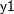from effect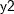, the regression method to impute variable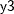from effectsand, the logistic regression method to impute variable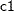from effects,, and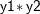, and the default regression method for continuous variables to impute variablefrom effects,, and:

```proc mi;
class c1;
fcs reg(y1= y2) reg(y3= y1 y2) logistic(c1= y1 y2 y1*y2);
var y1 y2 y3 c1;
run;
```